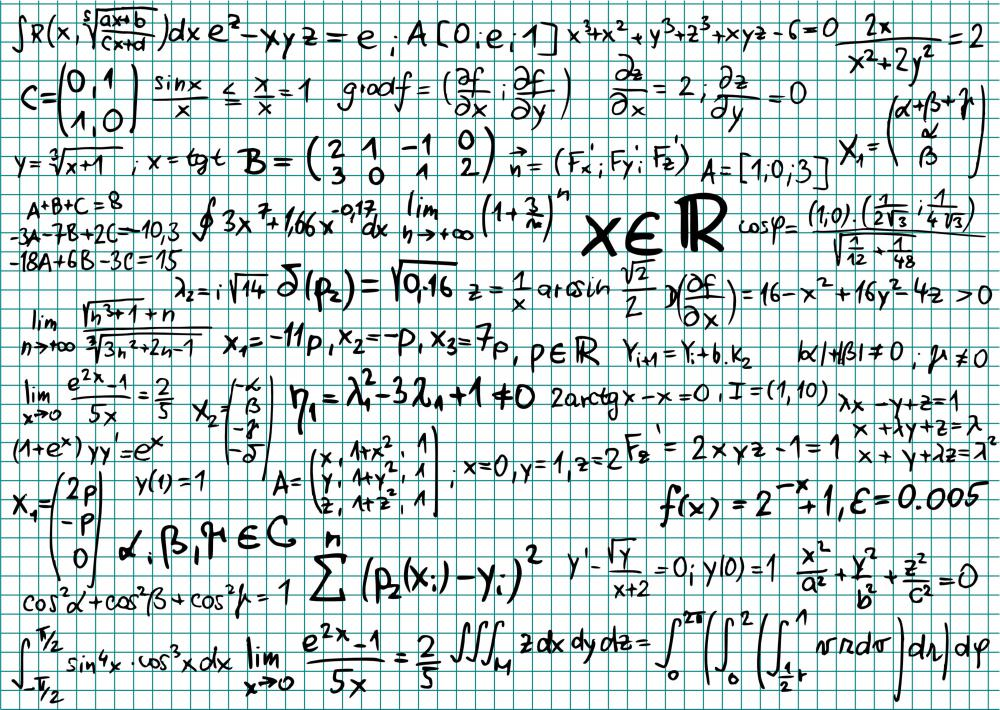# What is the Law of Large Numbers?

Ken Black

The law of large numbers is a statistical theorem postulating that the sample average of random variables will approach the theoretical average as the number of random variables increases. In other words, the larger a statistical sample is, the more likely one is to get results more accurate of the total picture. Lower sample numbers tend to skew the outcome more easily, though they can also be fairly accurate.Statistics and formulas are used because there is not enough time, or it is impractical, to use the entire population as a sample.

A coin is a good example that can be used to show the law of large numbers. Often, it is used in beginning-level statistics courses to demonstrate how effective this law can be. Most coins have two sides, heads and tails. If the coin is flipped, logic would say there are equal chances of the coin landing on the heads or tails side. Of course, this depends on the balance of the coin, its magnetic properties and other factors, but generally this is true.

If a coin is flipped only a few times, the results may not indicate there are equal chances of it landing on heads and tails. For example, flipping a coin four times may yield three heads and one tail. It could even yield four heads and no tails. This is a statistical anomaly.

However, the law of large numbers says that as the sample increases, those results will most likely fall in line with the true representation of the possibilities. If a coin is flipped 200 times, there is a good likelihood the number of times it lands on heads and tails will be near 100 each. However, the law or large numbers does not predict it will be exactly 100 each, only that it will likely be more representative of the true range of possibilities than a smaller average.

The law of large numbers demonstrates why an adequate sample is needed. Statistics are used because there is not enough time, or it is impractical, to use the entire population as a sample. However, a population sample means there will be representative members of the population that are not counted. In order to make sure the sample is reflective of the total population, an adequate number of random variables is needed.

Determining how large of a sample is needed normally depends on a number of factors, the main one being the confidence interval. For example, a statistical confidence interval is the level of certainty the population will fall within certain parameters. Setting a confidence interval of 95 percent would mean that there is a reasonable certainty 95 percent of the population will fall within those parameters. The sample needed for certain confidence intervals is determined by a formula that takes into account the number in the population as well as the confidence interval desired.

While the law of large numbers is a simple concept, the theorems and formulas that help justify it can be quite complex. Simply stated, the law or large numbers is the best explanation for why larger samples are better than smaller ones. No one can positively guarantee a statistical sampling will be completely accurate, but this law helps prevent many inaccurate results.

## You might also Likesubway11

I just wanted to say that whenever I think of the law of large number statistics I always think of the law of averages in sales.

It is well known that the more phone calls a salesperson makes the more appointments they would actually get. When I realized this when I worked in sales, I did not get caught up in the level of rejection that received over the phone.

I figured out that my average was about one appointment for every ten calls, so I anticipated nine rejections for every acceptance. If more people in sales realized this instead of trying to get every prospect to buy, more people would have a successful career in sales.